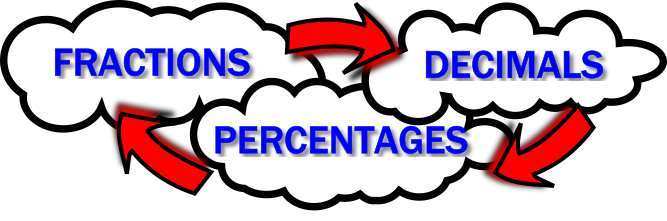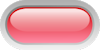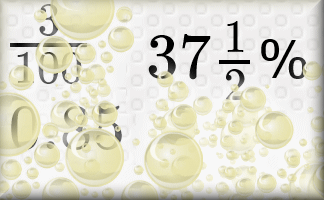#Fraction Decimal Percentage$$\frac12$$ $$0.5$$ $$50$$%$$\frac34$$ $$0.75$$ $$75$$%$$\frac14$$ $$0.25$$ $$25$$%$$\frac15$$ $$0.2$$ $$20$$%$$\frac35$$ $$0.6$$ $$60$$%$$\frac{11}{20}$$ $$0.55$$ $$55$$%$$\frac{7}{10}$$ $$0.7$$ $$70$$%$$\frac{1}{25}$$ $$0.04$$ $$4$$%$$\frac{3}{10}$$ $$0.3$$ $$30$$%$$\frac{3}{100}$$ $$0.03$$ $$3$$%$$\frac{17}{20}$$ $$0.85$$ $$85$$%$$\frac{1}{3}$$ $$0.\dot3$$ $$33\frac13$$%$$\frac{2}{3}$$ $$0.\dot6$$ $$66\frac23$$%$$\frac{1}{8}$$ $$0.125$$ $$12\frac12$$%$$\frac{3}{8}$$ $$0.375$$ $$37\frac12$$%$$\frac{7}{8}$$ $$0.875$$ $$87\frac12$$%$$\frac{5}{8}$$ $$0.625$$ $$62\frac12$$%$$\frac{1}{40}$$ $$0.025$$ $$2\frac12$$%$$\frac{17}{40}$$ $$0.425$$ $$42\frac12$$%$$\frac{7}{200}$$ $$0.035$$ $$3\frac12$$%$$\frac{7}{150}$$ $$0.04\dot6$$ $$4\frac23$$%## Copy and complete this table.

Teacher! You can customise this starter to suit your pupils. Click here to show all possible rows in the table above then click on the red buttons to hide the rows you don't want.

A short slide presentation is available to revise the operations needed to convert between fractions, decimals and percentages.

## A Mathematics Lesson Starter Of The Day

How did you use this starter? Can you suggest how teachers could present or develop this resource? Do you have any comments? It is always useful to receive feedback and helps make this free resource even more useful for Maths teachers anywhere in the world.
Click here to enter your comments.

Previous Day | This starter is for 27 July | Next Day

## Answers

Sign in to your Transum subscription account to see the answers

Your access to the majority of the Transum resources continues to be free but you can help support the continued growth of the website by doing your Amazon shopping using the links on this page. Below is an Amazon search box and some items I have chosen and recommend to get you started. As an Amazon Associate I earn a small amount from qualifying purchases which helps pay for the upkeep of this website.Teacher, do your students have access to computers?Do they have iPads or Laptops in Lessons? Whether your students each have a TabletPC, a Surface or a Mac, this activity lends itself to eLearning (Engaged Learning).Here a concise URL for a version of this page without the comments.

Transum.org/go/?Start=July27

Here is the URL which will take them to a related student activity.

Transum.org/go/?to=fdp## Curriculum Reference

See the National Curriculum page for links to related online activities and resources.For All:

©1997-2021 WWW.TRANSUM.ORG